public

General Purpose Bandgap Reference Circuit - avsdbgp_3v3_sky130_v2

A Bandgap Reference is an analog circuit, which is used to provide a constant output reference voltage being independent of Temperature, Process and Supply voltage variations. The analog IP avsdbgp_3v3 is a General Purpose Bandgap Reference ciruit, which is designed using Sky130nm technology in this project.

For detailed information regarding the Bandgap Reference circuit click here. The Design specifications provided for avsdbgp_3v3 can be found here.

• Performance parameters Bandgap Reference IP

• Circuit Implementation of Bandgap Reference IP

• Block Diagram of Bandgap Reference IP
• Schematic of Bandgap Reference IP

• Pre-Layout Simulation of Bandgap Reference IP circuit using Ngspice

• Ngspice
• Pre-Layout Simulation plots of Bandgap Reference IP circuit
• Vbgp v/s Temperature [ -40C - 140C] @ RL = 100M ohms plot
• Vbgp v/s VDD [ 2V - 4V] @ RL = 100M ohms plot
• Temperature Coefficient of Vbgp v/s Temperature [ -40C - 140C] @ RL = 100M ohms plot
• Voltage Coefficient of Vbgp v/s VDD [ 2V - 4V] @ RL = 100M ohms plot
• Start-Up Time of Vbgp @ RL = 100M ohms plot
• On-Off-Current of Vbgp wrt Enable @ RL = 100M ohms plot
• BGR Layout

• Magic
• Layout
• Post-Layout Simulation of Bandgap Reference IP circuit using Ngspice

• Vbgp v/s Temperature [ -40C - 140C] @ RL = 100M ohms plot
• Vbgp v/s VDD [ 2V - 4V] @ RL = 100M ohms plot
• Temperature Coefficient of Vbgp v/s Temperature [ -40C - 140C] @ RL = 100M ohms plot
• Voltage Coefficient of Vbgp v/s VDD [ 2V - 4V] @ RL = 100M ohms plot
• Start-Up Time of Vbgp @ RL = 100M ohms plot
• On-Off-Current of Vbgp wrt Enable @ RL = 100M ohms plot

Performance parameters of Bandgap Reference IP

Parameter Description Min Type Max Unit Condition
VBGP Output reference voltage 1.19490 1.19756 1.19764 Volt T= -40 to 140C, VDD=3.3V
VBGP Output reference voltage 1.11400 1.19756 1.21933 Volt VDD=2V to VDD=4V, T=27C
VDD Supply Voltage   3.3   Volt T=-40C to 125C
IDD Supply Current   21.9636   uA EN=1
IDD Supply Current   24.4   pA EN=0
RL Load Resistance   100   Mohm VDD=3.3V, T=27C

Circuit Implementation of Bandgap Reference IP

Block Diagram of Bandgap Reference IP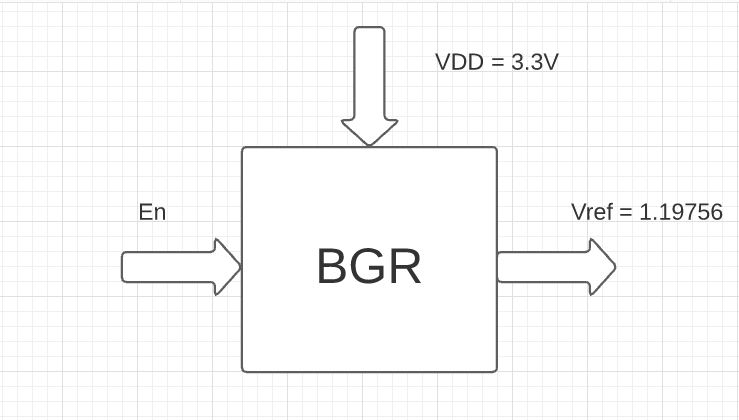Schematic of Bandgap Reference IP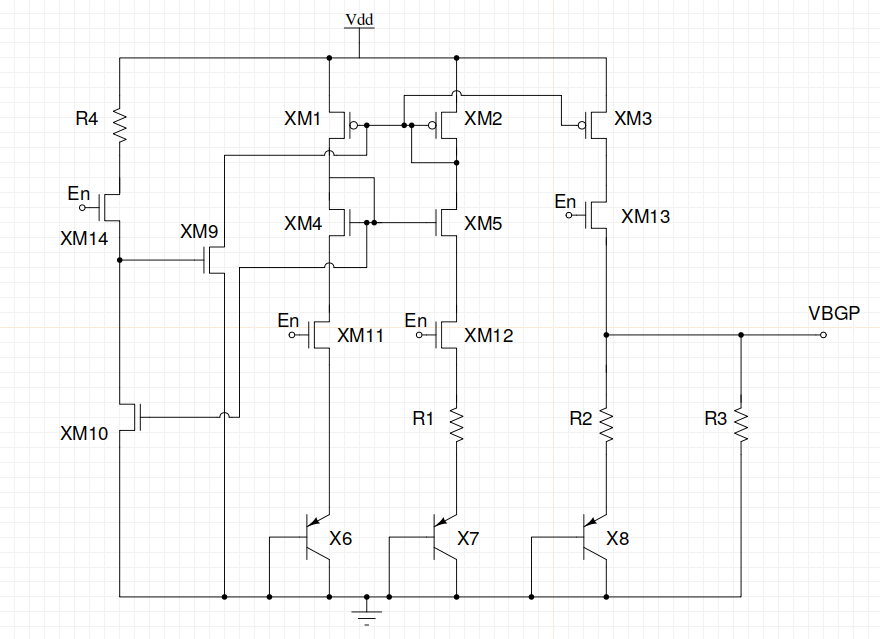The files from this repository can be downloaded and used by the following commands :-

sudo apt install -y git

git clone https://github.com/vsdip/avsdbgp_3v3_sky130_v2.git

Pre-Layout Simulation of Bandgap Reference IP circuit

The circuit implementation of Bandgap reference IP avsdbgp_3v3 is simulated using Ngspice to analyse its performance according to the Design Specifications provided.

Ngspice

Ngspice is an open source mixed-signal circuit simulator. To install Ngspice on Ubuntu, open terminal window and type :-

sudo apt-get install -y ngspice

After successful installation, to invoke Ngspice type the following command on the terminal window.

ngspice <circuit file to be simulated>

Pre- Layout Simulation plots of Bandgap Reference IP circuit

To simulate the files for Pre- Layout simulations

cd avsdbgp_3v3_sky130_v2/Pre_Layout_Simulations/Circuits/

Vbgp v/s Temperature [ -40C - 140C] @ RL = 100M ohms plot

To observe the effect of temperature on the circuit, the temperature is varied from -40C to 140C. On the terminal window, type :-

ngspice temp_avsdbgp_3v3.cir

The output plot as obtained can be seen below :-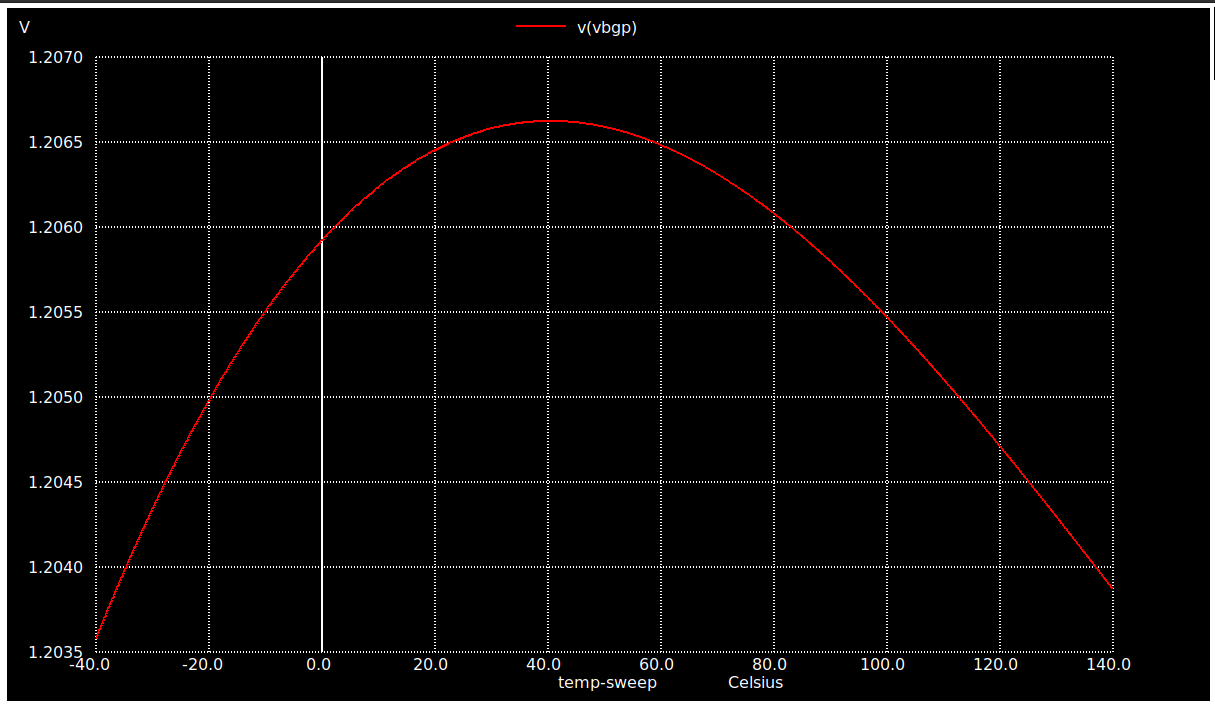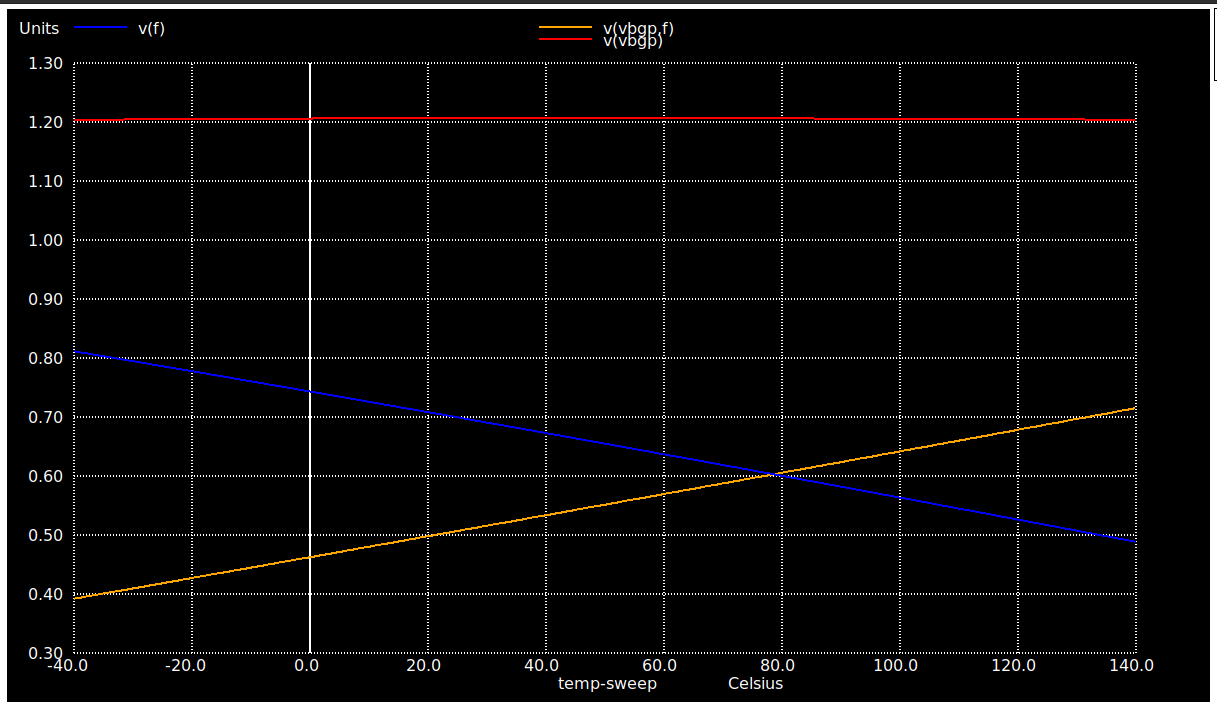Vbgp v/s VDD [ 2V - 4V] @ RL = 100M ohms plot

To observe the effect of Supply voltage on the circuit, the temperature is varied from 2V to 4V. On the terminal window, type :-

ngspice vdd_variation_avsdbgp_3v3.cir

The output plot as obtained can be seen below :-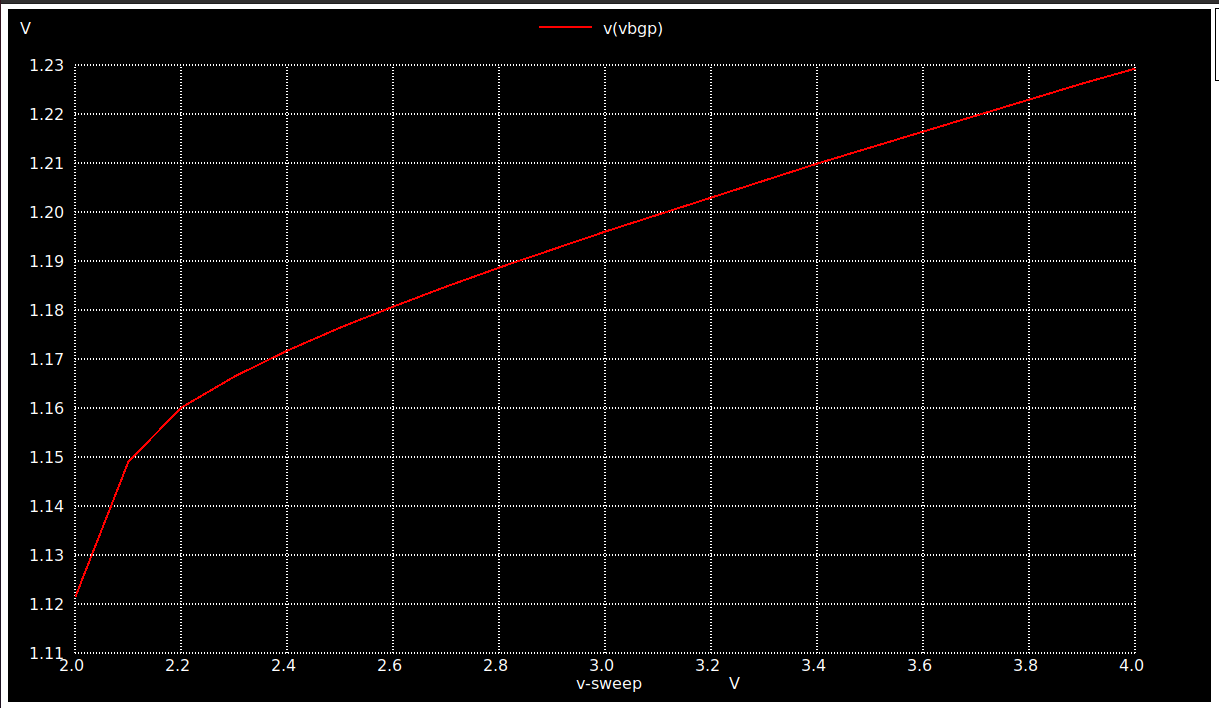Temperature Coefficient of Vbgp v/s Temperature [ -40C - 140C] @ RL = 100M ohms plot

On the terminal window, type :-

ngspice Temp_coeff_avsdbgp_3v3.cir

The output plot as obtained can be seen below :-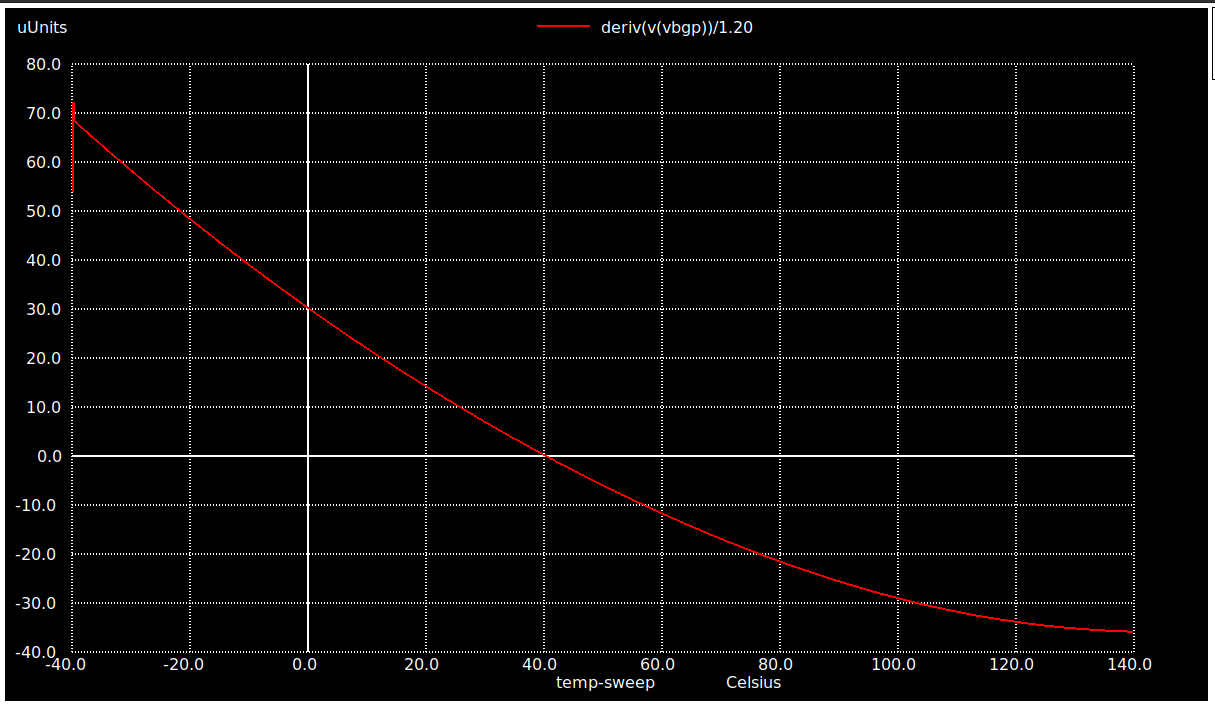Voltage Coefficient of Vbgp v/s VDD [ 2V - 4V] @ RL = 100M ohms plot

On the terminal window, type :-

ngspice voltage_coeff_avsdbgp_3v3.cir

The output plot as obtained can be seen below :-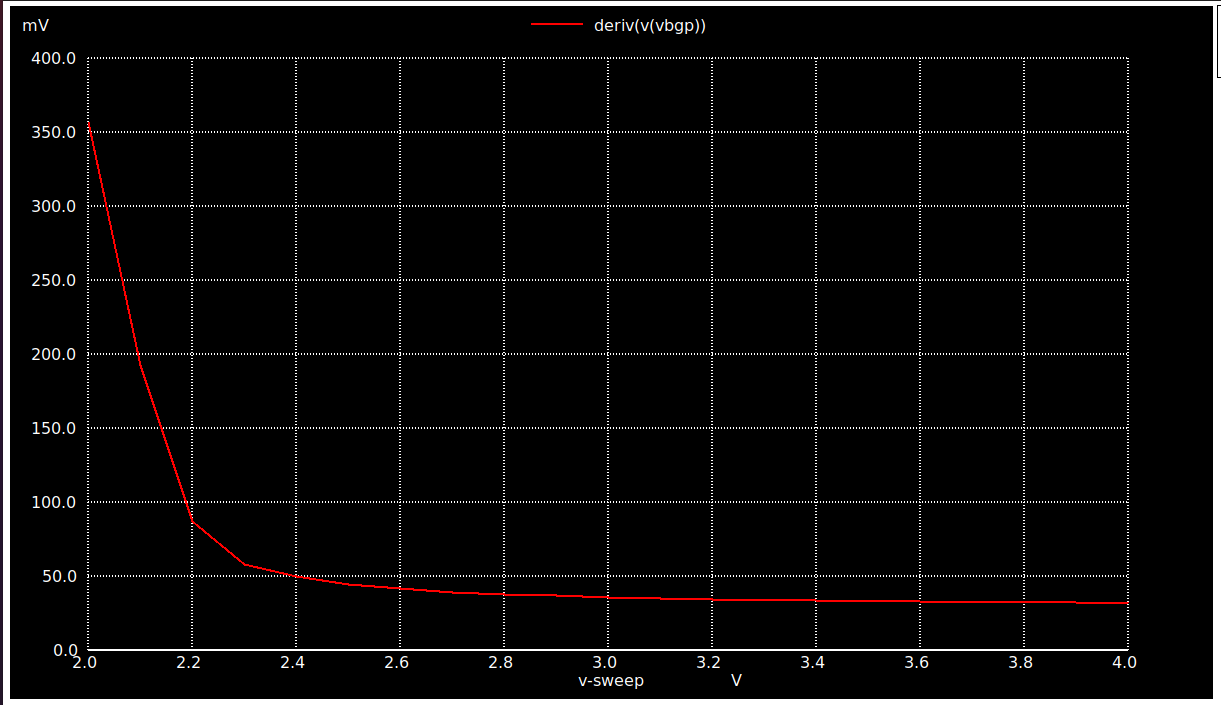Start-Up Time of Vbgp @ RL = 100M ohms plot

On the terminal window, type :-

ngspice Start_up_avsdbgp_3v3.cir

The output plot as obtained can be seen below :-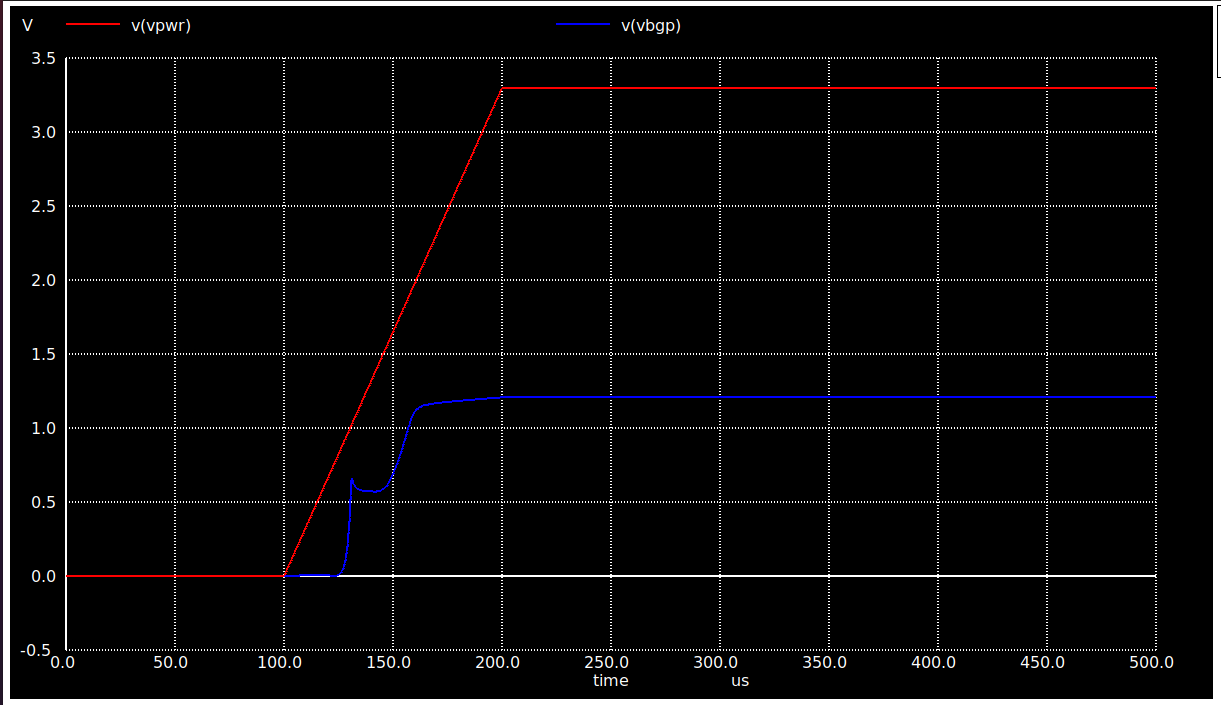On-Off-Current of Vbgp wrt Enable @ RL = 100M ohms plot

On the terminal window, type :-

ngspice enable_current.cir

The output plot as obtained can be seen below :-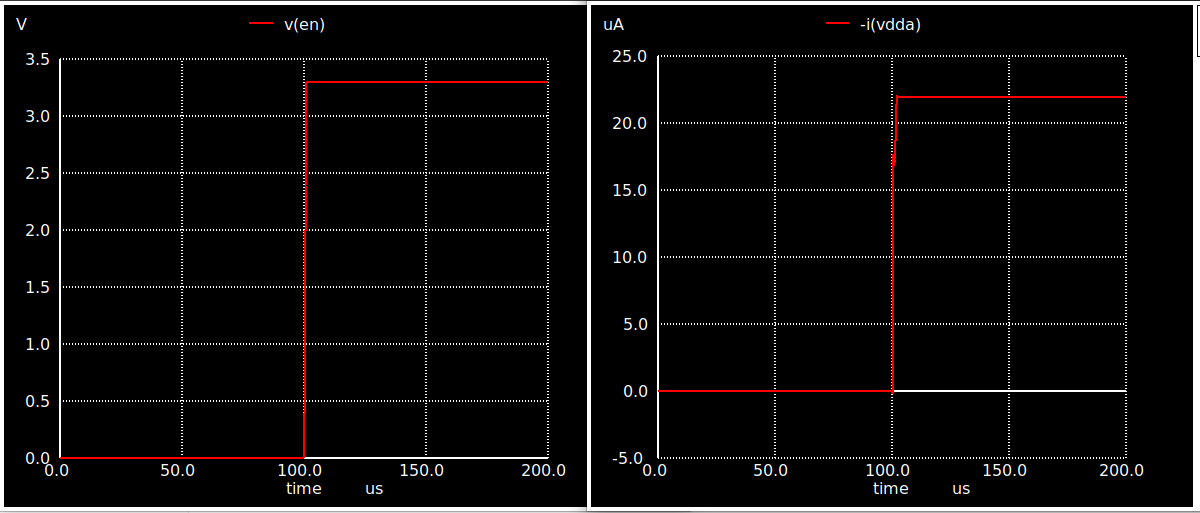BGR Layout

Magic

The Layout for the circuit was done using Magic Layout editor tool. To observe the layout, install magic using the following commands :-

sudo wget "http://opencircuitdesign.com/magic/archive/magic-8.3.122.tgz"

tar -xvzf magic-8.3.122.tgz

cd magic-8.3.122

sudo ./configure

sudo make

sudo make install

Layout

After successful installation, type:-

cd avsdbgp_3v3_sky130_v2/Layout/

magic -T ../libs/sky130A.tech BGR.mag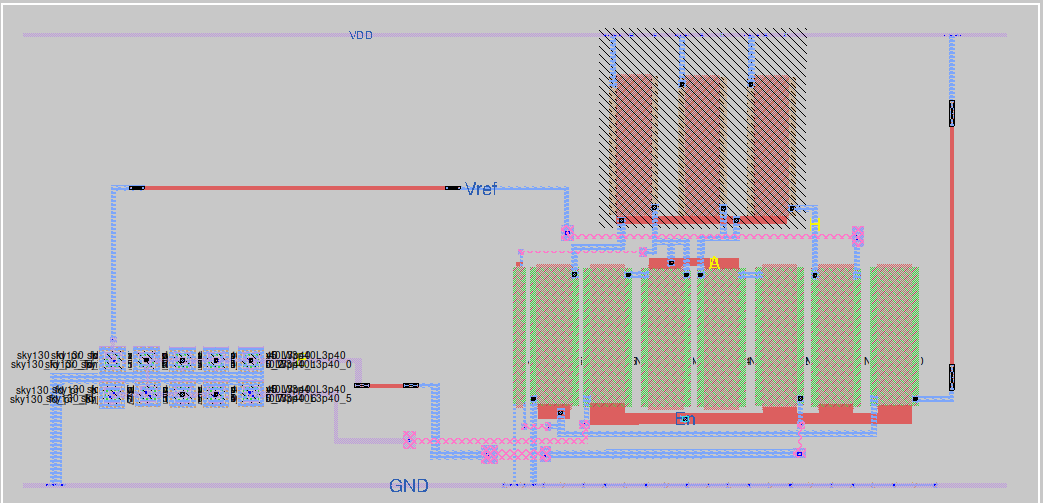Post-Layout Simulation plots of Bandgap Reference IP circuit

To simulate the files for Post-Layout simulations using ngspice :-

cd avsdbgp_3v3_sky130_v2/Post_Layout_Simulations/Circuits/

Vbgp v/s Temperature [ -40C - 140C] @ RL = 100M ohms plot

To observe the effect of temperature on the circuit, the temperature is varied from -40C to 140C. On the terminal window, type :-

ngspice temp_avsdbgp_3v3.spice

The output plot as obtained can be seen below :-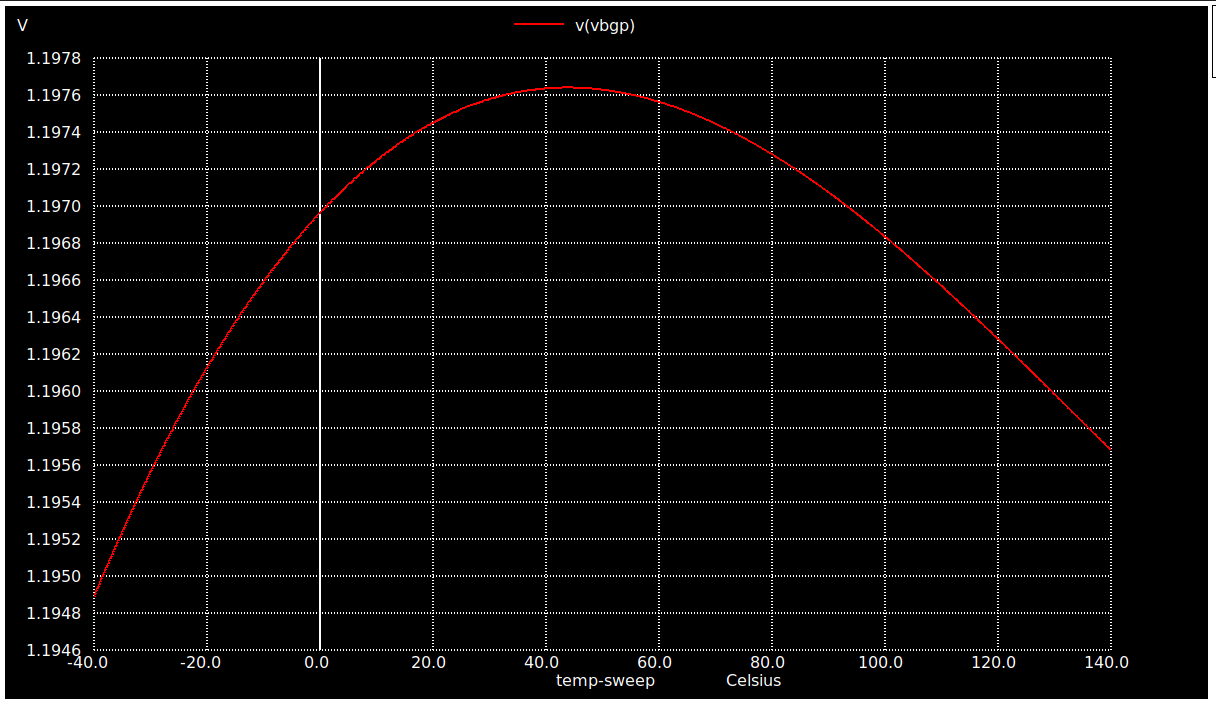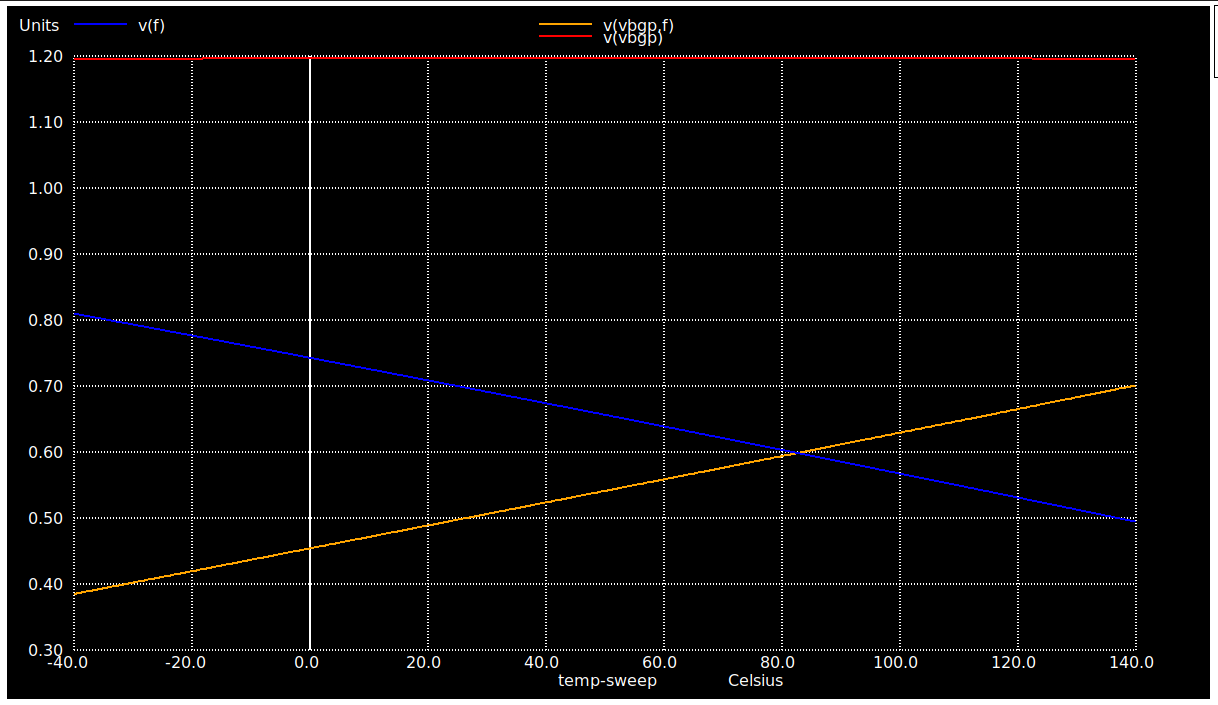Vbgp v/s VDD [ 2V - 4V] @ RL = 100M ohms plot

To observe the effect of Supply voltage on the circuit, the temperature is varied from 2V to 4V. On the terminal window, type :-

ngspice vdd_variation_avsdbgp_3v3.spice

The output plot as obtained can be seen below :-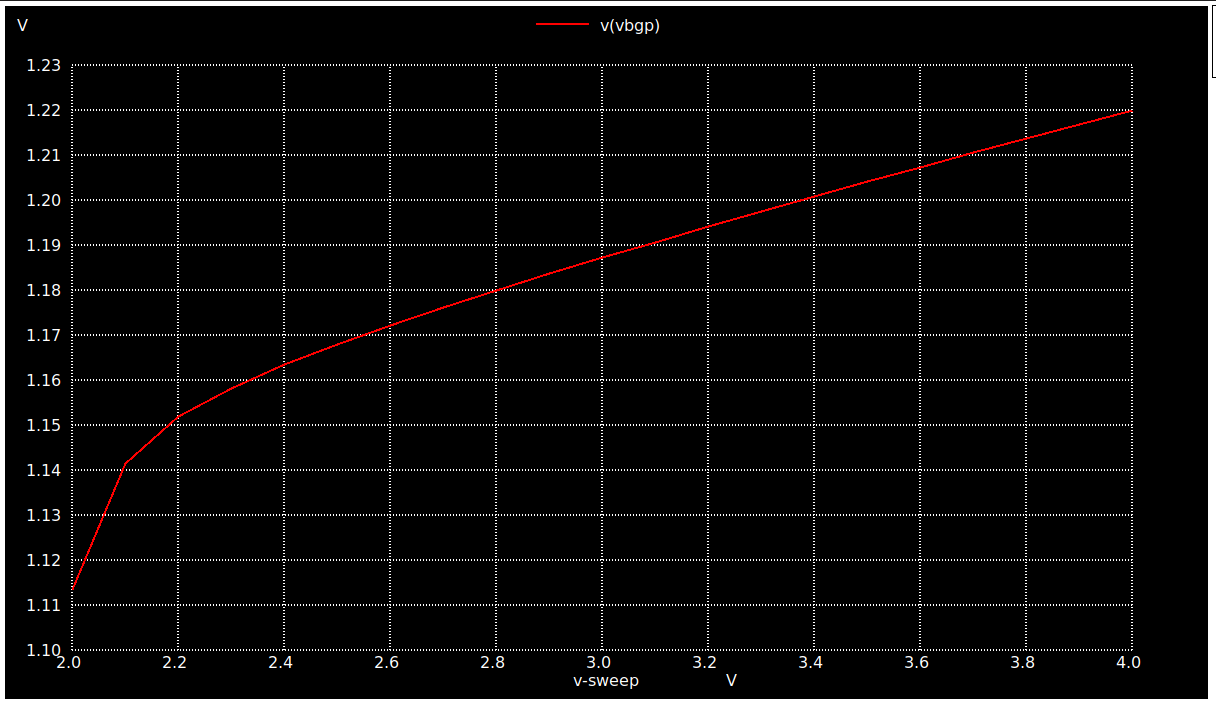Temperature Coefficient of Vbgp v/s Temperature [ -40C - 140C] @ RL = 100M ohms plot

On the terminal window, type :-

ngspice Temp_coeff_avsdbgp_3v3.spice

The output plot as obtained can be seen below :-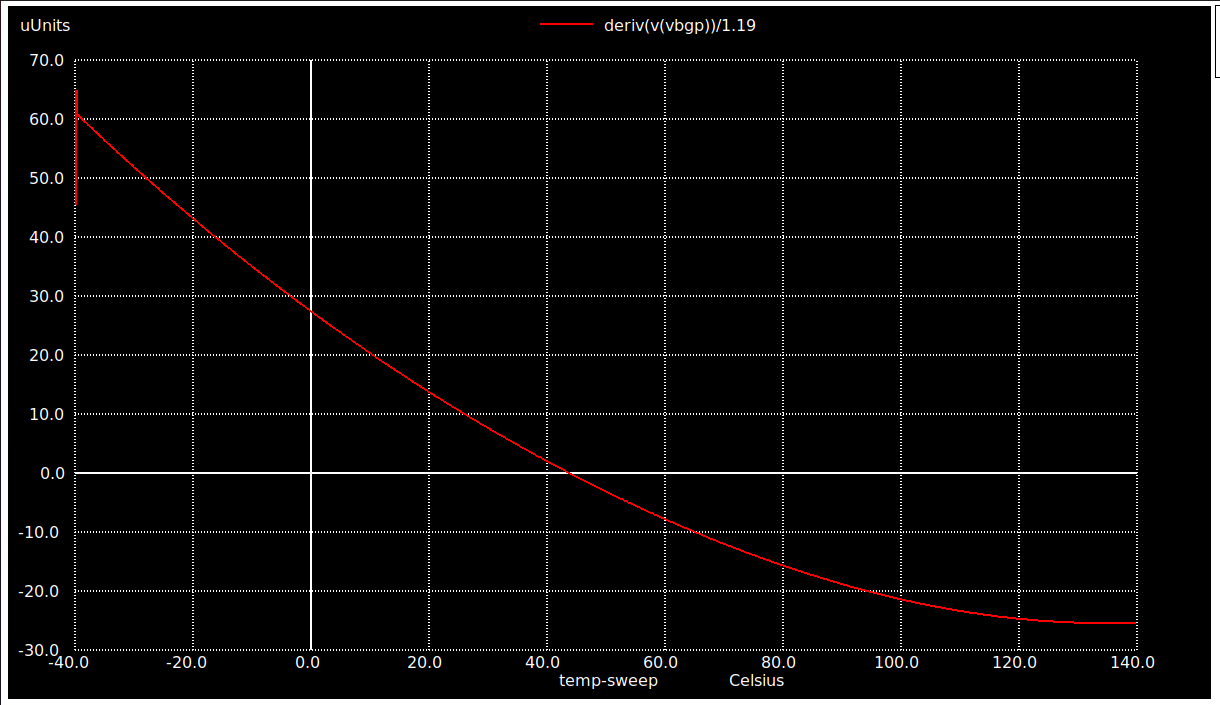Voltage Coefficient of Vbgp v/s VDD [ 2V - 4V] @ RL = 100M ohms plot

On the terminal window, type :-

ngspice voltage_coeff_avsdbgp_3v3.spice

The output plot as obtained can be seen below :-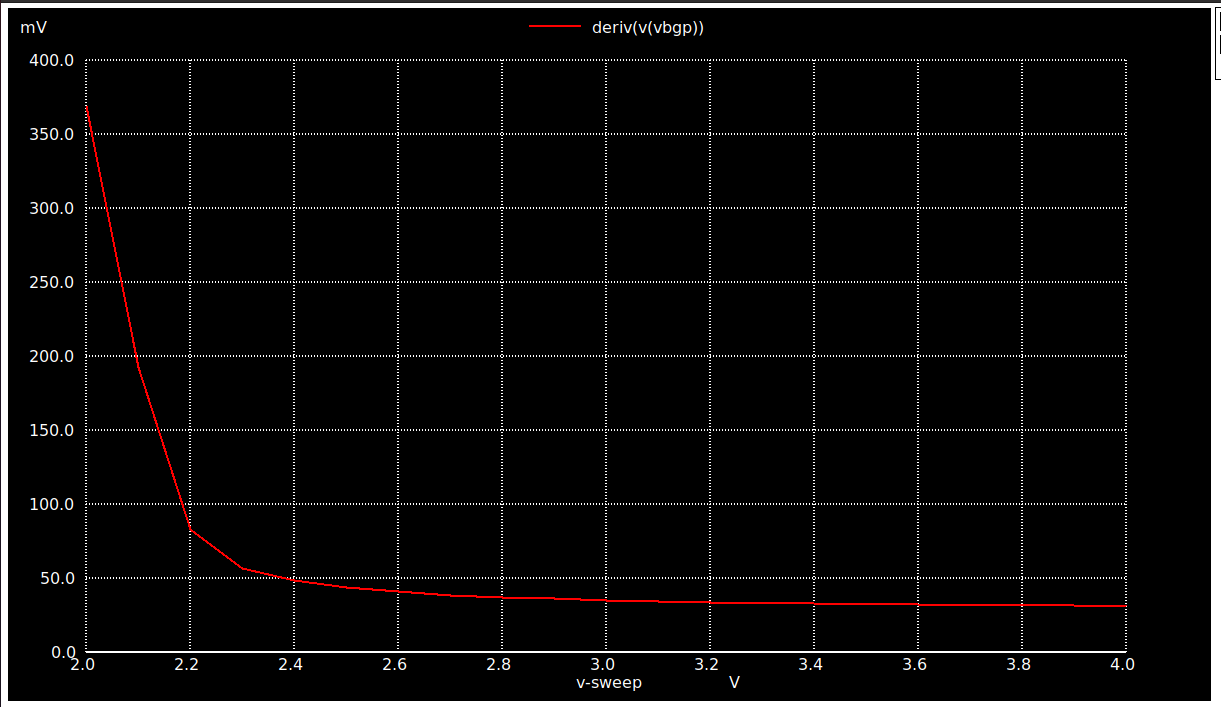Start-Up Time of Vbgp @ RL = 100M ohms plot

On the terminal window, type :-

ngspice Start_up_avsdbgp_3v3.spice

The output plot as obtained can be seen below :-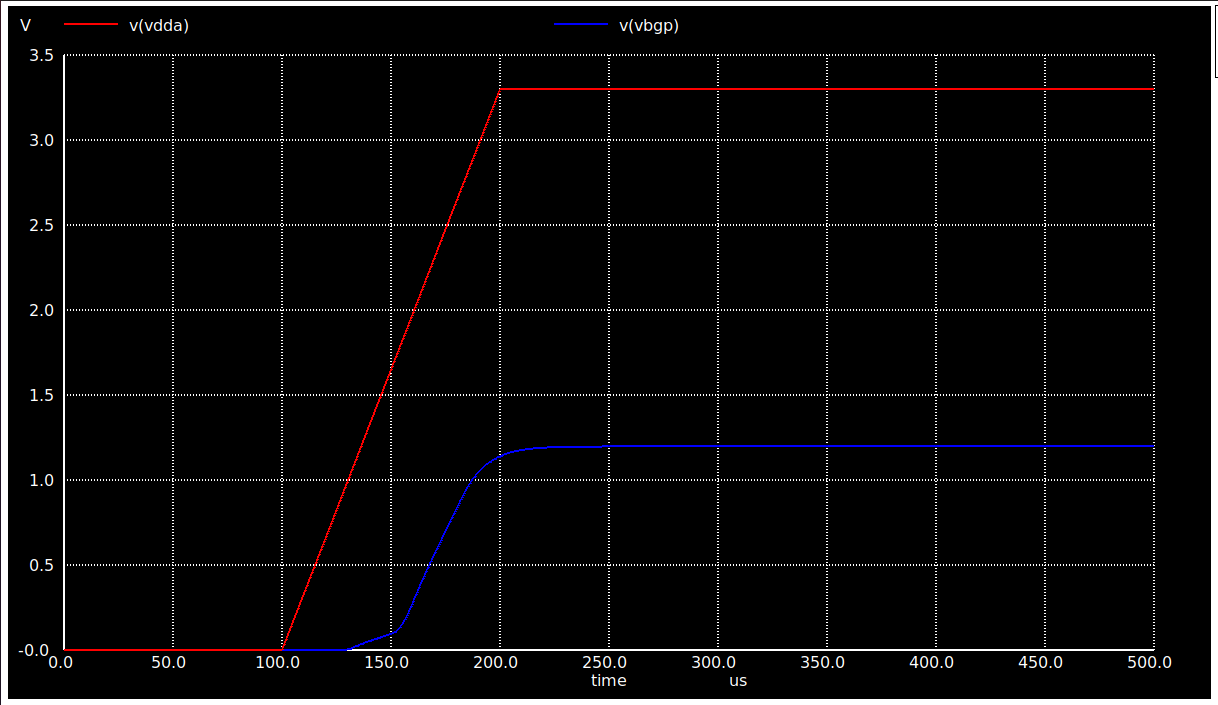Acknowledgements

• Kunal Ghosh, Co-founder of VLSI System Design (VSD) Corp. Pvt. Ltd.
• Tim Edwards, Senior Vice President of Analog and Design at efabless corporation.
• Sheryl Corina Serrao, Undergraduate Student, Mumbai University.

Contact Information

If you are collaborating on this project, please click here to access your collaboration files, and click "Accept Share" in the actions column if you haven't done so already.Layout Image

Anmol Purty

Summary

A General Purpose Bandgap Reference IP block that generates constant voltage at output, which is independent of Temperature and Supply variations.

bg

sky130A

MPW-TWO

Open MPW

Last MPW Precheck

Failed

12/11/21 09:48:37 PST